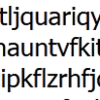#### You may also like### Route to Root

A sequence of numbers x1, x2, x3, ... starts with x1 = 2, and, if you know any term xn, you can find the next term xn+1 using the formula: xn+1 = (xn + 3/xn)/2 . Calculate the first six terms of this sequence. What do you notice? Calculate a few more terms and find the squares of the terms. Can you prove that the special property you notice about this sequence will apply to all the later terms of the sequence? Write down a formula to give an approximation to the cube root of a number and test it for the cube root of 3 and the cube root of 8. How many terms of the sequence do you have to take before you get the cube root of 8 correct to as many decimal places as your calculator will give? What happens when you try this method for fourth roots or fifth roots etc.?### Divided Differences

When in 1821 Charles Babbage invented the `Difference Engine' it was intended to take over the work of making mathematical tables by the techniques described in this article.### Probably a Code?

Is the regularity shown in this encoded message noise or structure?

# Procedure Solver

##### Age 16 to 18Challenge Level

Chanwc from Hong Kong, Matthew from QEB, Alpha Lee from Yew Chung International School, Phil from Garforth Community College and Andrei from Tudor Vianu National College all correctly worked out that the algorithm produces the greatest common factor of two whole numbers .

Aneesh correctly applied the algorithm for the cases X = 4 and Y = 1 and conjectured that the algorithm was to produce the output 1, whereas Alpha started with this example:

initial x_i = 25 y_i = 5
1. x_1 = 25-5 = 20 y_1 = 5 since x_i> y_i
2. x_2 = 20-5 =15 y_2 = 5 since x_1> y_1
3. x_3 = 15-5 =10 y_3 = 5 since x_2> y_2
4. x_4 =10-5 =5 y_4 = 5 since x_2> y_2
5. x = output = 5 since x_4 = y_4 gcd(25,5) = 5 since 25 = 5*5 5 = 5*1

The algorithm (which was discovered by the Greek mathematician Euclid over 2000 years ago) makes use of the fact that the greatest common divisor of two numbers (a ,b) equals the greatest common divisor of (a, b-a). Andre, Alpha and Phil all noticed this fact, giving good, clear proofs. Phil's notation was particularly clear :

Rename the two integers X and Y as AH and BH, meaning 'A multiples of the HCF' and 'B multiples of the HCF'. The difference between them, AH-BH, factorises to H(A-B). The difference between integers X and Y must be an integer, as must the difference between A and B, so the difference between X and Y must be a multiple of the HCF. Phil then noted that As the algorithm cycles, the numbers X and Y will always be replaced with lower multiples of the HCF of the original X and Y. The algorithm continues to subtract until only the HCF remains.

Matthew from QEB also solved the problem and, interestingly, converted the procedure into Javascript, a programming language most commonly used in webpages :

function getHCF(x,y) {
while (x != y) {
if (x > y) { x = x-y }
if (x < y) { y = y-x }
}
return x;
}

Matthew gave a clear and extended description of his code, which you can read about here .This is recommended for any budding programmers!